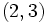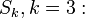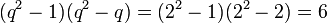# Difference between revisions of "Symmetric group:S3"

## Definition

### Verbal definitions

The symmetric group$S_3$ can be defined in the following equivalent ways:

### Multiplication table

We portray elements as permutations on the set$\{ 1,2,3 \}$ using the cycle decomposition. The row element is multiplied on the left and the column element on the right, with the assumption of functions written on the left. This means that the column element is applied first and the row element is applied next.

Element$()$$(1, 2)$$(2, 3)$$(1, 3)$$(1, 2, 3)$$(1, 3, 2)$$()$$()$$(1, 2)$$(2, 3)$$(1, 3)$$(1, 2, 3)$$(1, 3, 2)$$(1, 2)$$(1, 2)$$()$$(1, 2, 3)$$(1, 3, 2)$$(2, 3)$$(1, 3)$$(2, 3)$$(2, 3)$$(1, 3, 2)$$()$$(1, 2, 3)$$(1, 3)$$(1, 2)$$(1, 3)$$(1, 3)$$(1, 2, 3)$$(1, 3, 2)$$()$$(1, 2)$$(2, 3)$$(1, 2, 3)$$(1, 2, 3)$$(1, 3)$$(1, 2)$$(2, 3)$$(1, 3, 2)$$()$$(1, 3, 2)$$(1, 3, 2)$$(2, 3)$$(1, 3)$$(1, 2)$$()$$(1, 2, 3)$

If we used the opposite convention (i.e., functions written on the right), the row element is to be multiplied on the right and the column element on the left.

Here is the multiplication table where we use the one-line notation for permutations, where, as in the previous multiplication table, the column permutation is applied first and then the row permutation. Thus, with the left action convention, the row element is multiplied on the left and the column element on the right:

Element 123 213 132 321 231 312
123 123 213 132 321 231 312
213 213 123 231 312 132 321
132 132 312 123 231 321 213
321 321 231 312 123 213 132
231 231 321 213 132 312 123
312 312 132 321 213 123 231

VIEW RELATED: Analogues of this | Variations of this | Opposites of this |[SHOW MORE]
View a complete list of particular groups (this is a very huge list!)[SHOW MORE]

## Families

The symmetric group on three elements is part of some important families:

Generic name for family member Definition Parametrization of family Parameter value(s) for this member Other members Comments
symmetric group on finite set group of all permutations on a finite set by a nonnegative integer, denoting size of set acted on 3 click here for a list
Coxeter group has a presentation of a particular form Coxeter matrix describing the presentation click here for a list symmetric groups on finite sets are Coxeter groups
dihedral group semidirect product of a cyclic group and a two-element group acting via the inverse map by a positive integer that's half the order 3 click here for a list
general linear group general linear group of finite degree over a finite field name of field, degree field:F2 (size two), degree two click here for a list
projective general linear group projective general linear group of finite degree over a finite field name of field, degree field:F2 (size two), degree two click here for a list
special linear group special linear group of finite degree over a finite field name of field, degree field:F2 (size two), degree two click here for a list
projective special linear group projective special linear group of finite degree over a finite field name of field, degree field:F2 (size two), degree two click here for a list

## Elements

Further information: Element structure of symmetric group:S3

### Up to conjugacy

As for any symmetric group, cycle type determines conjugacy class. The cycle types, in turn, are parametrized by the unordered integer partitions of$3$. The conjugacy classes are described below.

Partition Partition in grouped form Verbal description of cycle type Elements with the cycle type in cycle decomposition notation Elements with the cycle type in one-line notation Size of conjugacy class Formula for size Even or odd? If even, splits? If splits, real in alternating group? Element order Formula calculating element order
1 + 1 + 1 1 (3 times) three fixed points$()$ -- the identity element 123 1$\! \frac{3!}{(1)^3(3!)}$ even; no 1$\operatorname{lcm} \{ 1, 1, 1 \}$
2 + 1 2 (1 time), 1 (1 time) transposition in symmetric group:S3: one 2-cycle, one fixed point$(1,2)$,$(1,3)$,$(2,3)$ 213, 321, 132 3$\! \frac{3!}{(2)(1)}$ odd 2$\operatorname{lcm} \{ 2,1 \}$
3 3 (1 time) 3-cycle in symmetric group:S3: one 3-cycle$(1,2,3)$,$(1,3,2)$ 231, 312 2$\! \frac{3!}{3}$ even; yes; no 3$\operatorname{lcm} \{ 3 \}$
Total (3 rows -- 3 being the number of unordered integer partitions of 3) -- -- -- -- 6 (equals 3!, the size of the symmetric group) -- odd: 3
even;no: 1
even; yes; no: 2
order 1: 1, order 2: 3, order 3: 2 --

This group is one of three finite groups with the property that any two elements of the same order are conjugate. The other two are the cyclic group of order two and the trivial group.

### Up to automorphism

The classification of elements upto automorphism is the same as that upto conjugation; this is because the symmetric group on three elements is a complete group: a centerless group where every automorphism is inner.

Enter quick page feedback right here, without leaving the page: [SHOW MORE]

## Arithmetic functions

### Basic arithmetic functions

Function Value Similar groups Explanation
order (number of elements, equivalently, cardinality or size of underlying set) 6 groups with same order As symmetric group$\! S_k, k = 3:$,$\! k! = 3! = 3 \cdot 2 \cdot 1 = 6$

as general linear group of degree two$\! GL(2,q), q = 2:$$\! (q^2 - 1)(q^2 - q) = (2^2 - 1)(2^2 - 2) = 6$
exponent of a group 6 groups with same order and exponent of a group | groups with same exponent of a group Elements of order$2$ and$3$.
derived length 2 groups with same order and derived length | groups with same derived length Cyclic subgroup of order three is abelian, has abelian quotient.
minimum size of generating set 2 groups with same order and minimum size of generating set | groups with same minimum size of generating set$(1,2), (1,2,3)$
subgroup rank of a group 2 groups with same order and subgroup rank of a group | groups with same subgroup rank of a group All proper subgroups are cyclic.
max-length of a group 2 groups with same order and max-length of a group | groups with same max-length of a group] Subgroup series going through subgroup of order two or three.

### Arithmetic functions of an element-counting nature

Function Value Similar groups Explanation
number of conjugacy classes 3 groups with same order and number of conjugacy classes | groups with same number of conjugacy classes The three classes are the identity element, the transpositions, and the 3-cycles.
number of equivalence classes under real conjugacy 3 groups with same order and number of equivalence classes under real conjugacy | groups with same number of equivalence classes under real conjugacy Same as the number of conjugacy classes, because the group is an ambivalent group.
number of conjugacy classes of real elements 3 groups with same order and number of conjugacy classes of real elements | groups with same number of conjugacy classes of real elements Same as the number of conjugacy classes, because the group is an ambivalent group.
number of equivalence classes under rational conjugacy 3 groups with same order and number of equivalence classes under rational conjugacy | groups with same number of equivalence classes under rational conjugacy Same as the number of conjugacy classes, because the group is a rational group.
number of conjugacy classes of rational elements 3 groups with same order and number of conjugacy classes of rational elements | groups with same number of conjugacy classes of rational elements Same as the number of conjugacy classes, because the group is an ambivalent group.

### Arithmetic functions of a subgroup-counting nature

Function Value Similar groups Explanation
number of subgroups 6 See subgroup structure of symmetric group:S3
number of conjugacy classes of subgroups 4
number of normal subgroups 3 groups with same order and number of normal subgroups | groups with same number of normal subgroups
number of automorphism classes of subgroups 4

### Lists of numerical invariants

List Value Explanation/comment
conjugacy class sizes$1,2,3$ See cycle type determines conjugacy class, element structure of symmetric group:S3, element structure of symmetric groups
order statistics$1 \mapsto 1, 2 \mapsto 3, 3 \mapsto 2$
degrees of irreducible representations$1,1,2$ See linear representation theory of symmetric group:S3, linear representation theory of symmetric groups
orders of subgroups$1,2,2,2,3$ See subgroup structure of symmetric group:S3

## Group properties

### Important properties

Property Satisfied? Explanation Comment
Abelian group No$(1,2)$ and$(2,3)$ don't commute Smallest non-abelian group
Nilpotent group No Centerless: The center is trivial Smallest non-nilpotent group
Metacyclic group Yes Cyclic normal subgroup of order three, cyclic quotient of order two
Supersolvable group Yes Metacyclic implies supersolvable
Solvable group Yes Metacyclic implies solvable

## Subgroups

Further information: Subgroup structure of symmetric group:S3

There are six subgroups:

1. The identity element is the trivial subgroup (1)
2. S2 in S3: There are three 2-element subgroups, generated by the transpositions. These are all conjugate subgroups, and each is isomorphic to the cyclic group of order two (3)
3. A3 in S3: There is one 3-element subgroup, generated by a 3-cycle. This is a characteristic subgroup, and is isomorphic to the cyclic group of order three. This is, concretely, the alternating group on three letters (i.e., the group of even permutations on three letters). (1)
4. The whole group (1)

### Normal subgroups

There are three normal subgroups: the trivial subgroup (type (1)), the three-element subgroup (type (3)), and the whole group (type (4)). It turns out that these are also the same as the characteristic subgroups and the same as the fully characteristic subgroups.

### Sylow subgroups

• There is a unique (normal) 3-Sylow subgroup: the 3-element subgroup (type (3) in the list).
• There are three 2-Sylow subgroups: the three 2-element subgroups generated by transpositions (type (2) in the list).

The Sylow subgroups in this group enjoy two special properties:

• Every element in the symmetric group lies inside one of the Sylow subgroups
• Every proper nontrivial subgroup is a Sylow subgroup.

Further information: Subgroup structure of symmetric group:S3

Enter quick page feedback right here, without leaving the page: [SHOW MORE]

## Subgroup-defining functions

Subgroup-defining function Subgroup type in list Isomorphism class Page on subgroup Comment
center (1) Trivial group -- The group is centerless
derived subgroup (3) cyclic group:Z3 A3 in S3
Frattini subgroup (1) trivial group -- The$3$-Sylow and$2$-Sylow are maximal and intersect trivially
socle (3) Cyclic group of order three A3 in S3 This subgroup is the unique minimal normal subgroup, i.e.,the monolith, and the group is monolithic.

## Quotient-defining functions

Quotient-defining function Isomorphism class Comment
Inner automorphism group Symmetric group:S3 It is the quotient by the center, which is trivial.
Abelianization Cyclic group of order two It is the quotient by the commutator subgroup, which is cyclic of order three.

## Distinguishing features

### Smallest of its kind

• This is the unique non-abelian group of smallest order. All groups of order up to$5$, and all other groups of order$6$, are abelian.
• This is the unique non-nilpotent group of smallest order. All groups of order up to$5$, and all other groups of order$6$, are nilpotent.
• This is the unique smallest nontrivial complete group.

## Other associated constructs

Associated construct Isomorphism class Comment
automorphism group symmetric group:S3 the group is a complete group, hence is isomorphic to its automorphism group. See also symmetric groups are complete
extended automorphism group direct product of S3 and Z2
quasiautomorphism group direct product of S3 and Z2
outer automorphism group trivial group the group is a complete group, hence its outer automorphism group is trivial.
1-automorphism group direct product of S3 and Z2
holomorph direct product of S3 and S3

## GAP implementation

### Group ID

This finite group has order 6 and has ID 1 among the groups of order 6 in GAP's SmallGroup library. For context, there are groups of order 6. It can thus be defined using GAP's SmallGroup function as:

SmallGroup(6,1)

For instance, we can use the following assignment in GAP to create the group and name it$G$:

gap> G := SmallGroup(6,1);

Conversely, to check whether a given group$G$ is in fact the group we want, we can use GAP's IdGroup function:

IdGroup(G) = [6,1]

or just do:

IdGroup(G)

to have GAP output the group ID, that we can then compare to what we want.

### Other descriptions

Description Functions used
SymmetricGroup(3) SymmetricGroup
DihedralGroup(6) DihedralGroup
GL(2,2) GL
Enter quick page feedback right here, without leaving the page: [SHOW MORE]